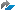Show TOC

# Internal Rules for Update StatisticsUse

This algorithm is used by BRCONNECT to update statistics. For more information, see Update Statistics with BRCONNECT.

1. BRCONNECT determines the working set of tables and indexes to be checked and updated. To do this, it uses:

2. If the working set contains pool, cluster (for Oracle 9i only) or other tables that have the ACTIVE flag in the DBSTATC table set to N, BRCONNECT immediately deletes the statistics for these tables. This is because they negatively affect database performance.

3. BRCONNECT checks statistics for the remaining tables in the working set, including tables that have the ACTIVE flag in the DBSTATC table set to A, P, or R as follows:

• If the table has the MONITORING attribute set, BRCONNECT reads the number of inserted, deleted, and updated rows from the DBA_TAB_MODIFICATIONS table (this is standard in Oracle 10g or higher).

• Otherwise, BRCONNECT uses the standard method (see table below) to update statistics by using the unique index.

Note

BRCONNECT uses the following standard method to check and update a table's statistics:

• Method and sample defined for the table in the DBSTATC table (has highest priority)

• Method and sample from the options -m|-method or -s|-sample of -f stats -method (takes priority) or the stats_method and stats_sample_size parameters

• Default method and sample (has lowest priority)

The following table describes the default method:

Number of Rows in Table

Analysis Method

Sample Size

Rows

< 10,000

C

10,000 <=

Rows

< 100,000

E

P30

100,000 <=

Rows

< 1,000,000

E

P10

1,000,000 <=

Rows

< 10,000,000

E

P3

10,000,000 <=

Rows

< 100,000,000

E

P1

100,000,000 <=

Rows

< 1,000,000,000

E

P.3

1,000,000,000 <=

Rows

< 10,000,000,000

E

P.1

10,000,000,000 <=

Rows

< 100,000,000,000

E

P.03

100,000,000,000 <=

Rows

< 1,000,000,000,000

E

P.01

1,000,000,000,000 <=

Rows

< 10,000,000,000,000

E

P.003

10,000,000,000,000 <=

Rows

E

P.001

Analysis method C means compute the statistics exactly. Analysis method E means estimate the statistics using the sample size specified.

For example, “E P10” means that BRCONNECT takes an estimated sample using 10% of rows.

For the CH, CX, EH, and EX methods, histograms are created.

For the CI, CX, EI and EX methods, the structure of indexes is validated in addition to collecting statistics. However, this locks the indexes.

4. BRCONNECT uses the number of new rows for each table in the working set, as derived in the previous step, to see if either of the following is true:

• If table MONITORING is used (standard in Oracle 10g or higher):

• #old rows + # inserted rows >= # old rows * (100 + threshold) / 100

• #old rows + # updated rows >= # old rows * (100 + threshold) / 100

• #old rows - # deleted rows <= # old rows * 100 / (100 + threshold)

• If table MONITORING is not used:

• Number of new rows is greater than or equal to number of old rows * (100 + threshold) / 100

• Number of new rows is less than or equal to number of old rows * 100 / (100 + threshold)

The standard threshold is 50, but the value defined in -f stats -change or the stats_change_threshold parameter is used if specified.

5. BRCONNECT immediately updates statistics after checking for the following tables:

• Tables where either of the conditions in the previous step is true

• Tables from the DBSTATC table with the following value:

ACTIVE field U (unconditional)

6. BRCONNECT writes the results of update statistics to the DBSTATTORA table and also, for tables with the DBSTATC history flag or usage type A, to the DBSTATHORA table.

7. For tables with update statistics using methods EI, EX, CI, or CX, BRCONNECT validates the structure of all associated indexes and writes the results to the DBSTATIORA table. BRCONNECT also does this for tables with the DBSTATC history flag or usage type A, writing the results to the DBSTAIHORA table.

For more information about special rules for updating statistics of individual table partitions, see SAP Notes 744483and 865366.